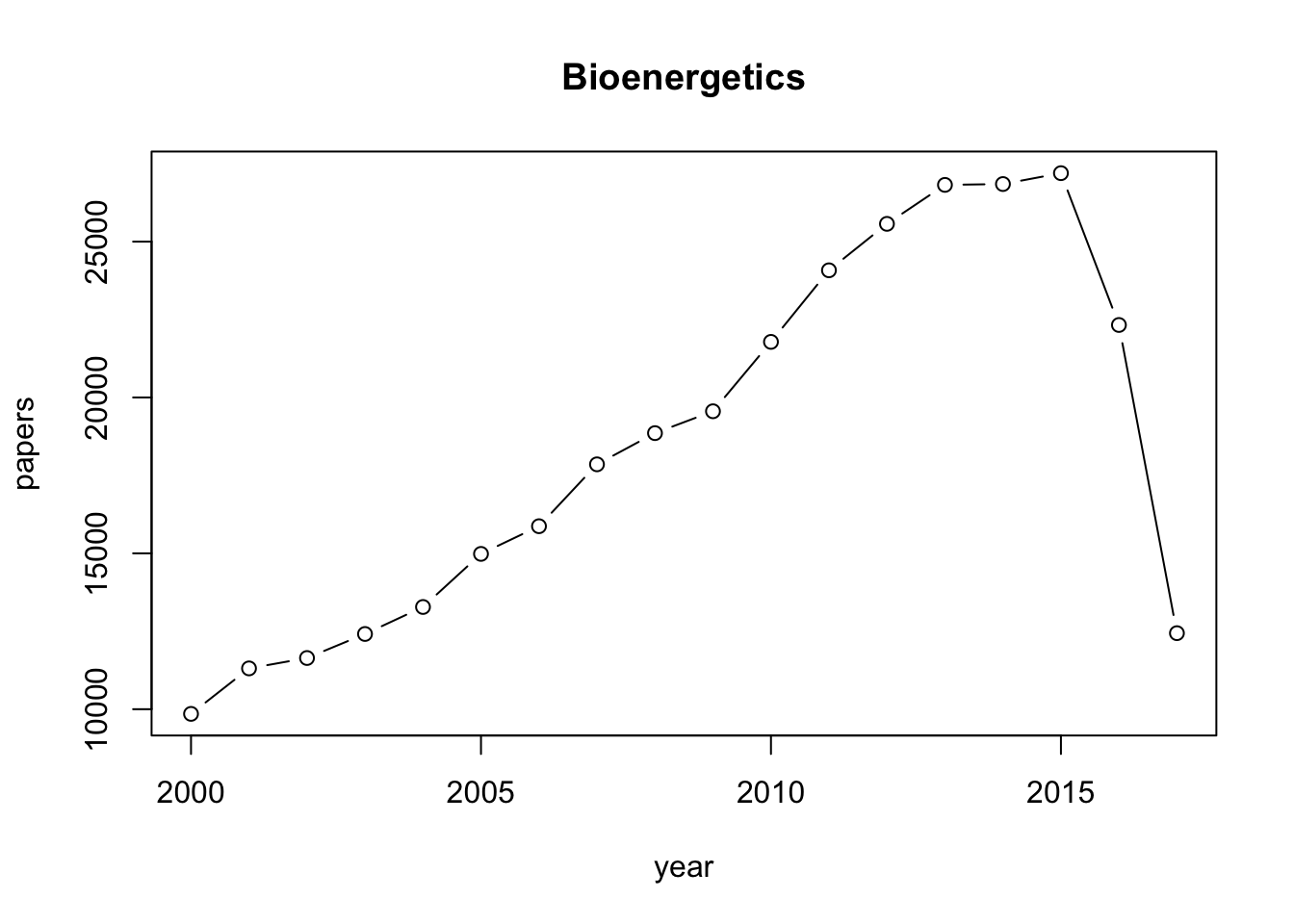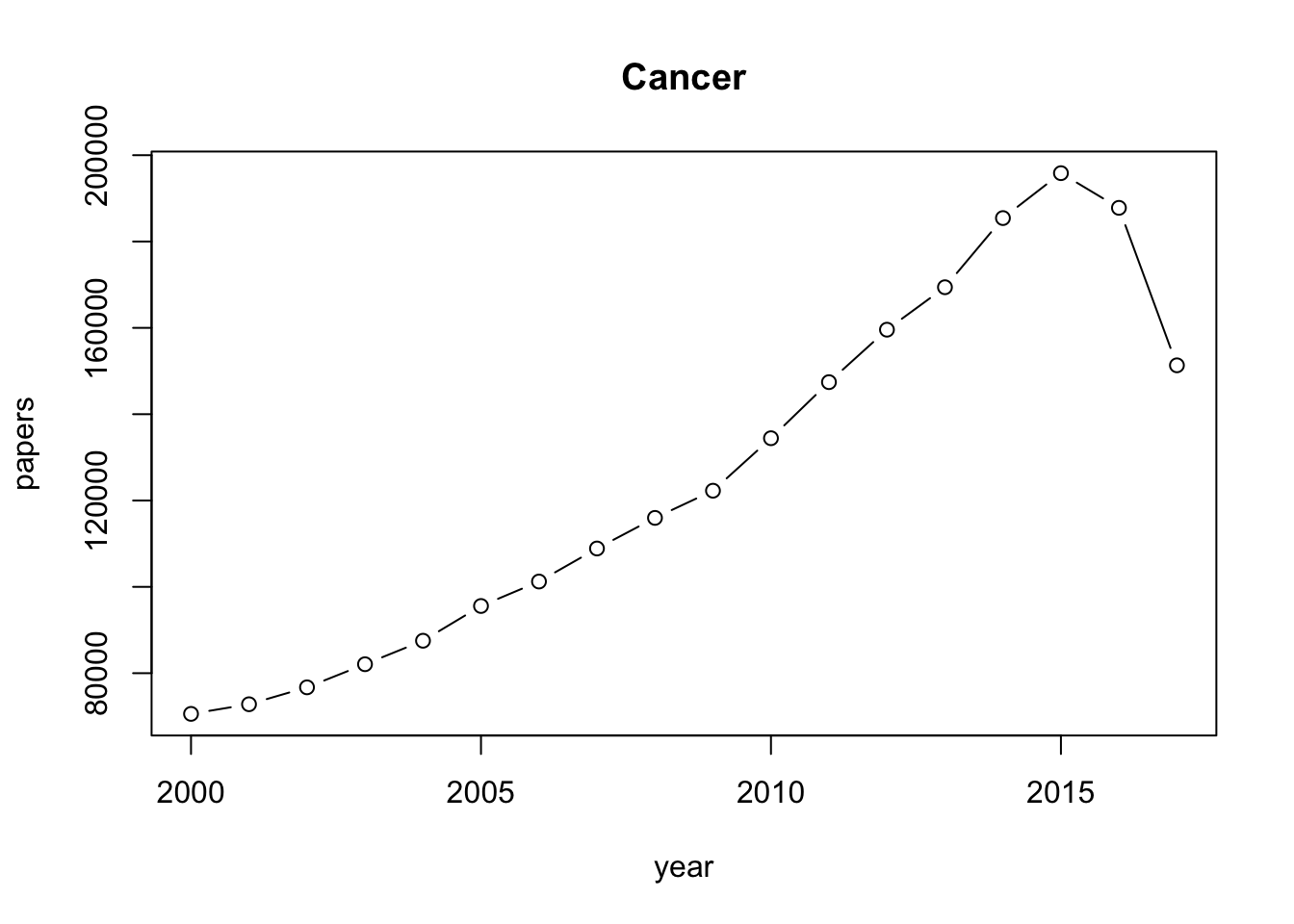library("rentrez")
#term <- readline(prompt="Enter search term ")
#year1 <- as.integer(year1)
term<-"Bioenergetics"
year1<-2000
year2<-2017
#year2<- as.integer(year2)

year<-year1:year2
search_year <- function(year, term){
query <- paste(term, "AND (", year, "[PDAT])")
entrez_search(db="pubmed", term=query, retmax=0)$count } papers <- sapply(year, search_year, term, USE.NAMES=FALSE) plot(year, papers, type='b', main=term)term<- "Cancer" year1<-2000 year2<-2017 #year2<- as.integer(year2) year<-year1:year2 search_year <- function(year, term){ query <- paste(term, "AND (", year, "[PDAT])") entrez_search(db="pubmed", term=query, retmax=0)$count
}
papers <- sapply(year, search_year, term, USE.NAMES=FALSE)
plot(year, papers, type='b', main=term)## Equilibrium constant and free energy in correct forms

The Gibbs free energy change due to ATP hydrolysis depends upon the concentrations of the various participants in the reactions. When the concentrations are farther from their equilibrium values, the absolute value of free energy is greater. Explain
Under “standard” conditions (i.e. concentrations of 1M for all reactants except water which is taken at its characteristic concentration of 55M) the Gibbs free energy of ATP hydrolysis varies from -28 to -34 kJ/mol (i.e. ≈12 kBT, BNID 101989) depending on the concentration of the cation Mg2+.
The dependence on Mg ions occurs because the positively-charged magnesium ions help to stabilize the ATP molecule.

However, in the cell the conditions are never even close to the standard state values. For example, a concentration of 1M ATP would mean that the mass of solute would be similar to that of the water solvent itself.
Often confusing derivation of the physiological free energy is given the ratio of concentrations from the standard value. The division by terms such as [1M] are required in order to take care of units as a logarithm should always contain a unitless term.
It is sometimes surprising to think that if the cell was at equilibrium, the value of free enerhy would have been zero, and there would be no energy to gain by ATP hydrolysis. *Fortunately, this is never the case in living organisms.

With this in mind let us express the free energy of ATP hydrolysis under standard conditions in the correct format. $K'_{eq} = \frac{[ADP]_{eq}/[1M]\cdot [Pi]_{eq}/[1M]}{[ATP]_{eq}/[1M]\cdot [H_2O]/[55M]}\\ \Delta G'_o= -RT ln K'_{eq}=(35- 40)\frac{kJ}{Mol}$

In the physiological condition the mass action ratio will be given by : $Q=\frac{[ADP]_{physiol}/[1M]\cdot [Pi]_{physiol}/[1M]}{[ATP]_{physiol}/[1M]\cdot [H_2O]/[55M]}\\ \Delta G = \Delta G'_o + RT ln Q = (-50 , -70) \frac{kJ}{Mol}$ In practice the physiological conditions depend on the organism being studied, the tissue or compartment within the cell under consideration, and on the current energy demands for metabolic and other reactions. For example, in perfused rat liver the ATP to ADP ratio was found to be about 10:1 in the cytosol but 1:10 in the mitochondria under high rates of glycolysis, and under low rates of glycolysis both ratios were much close to 1 (BNID 111357).
Therefore a range of values for $$\Delta G$$ is expected. The key to understanding this range is to get a sense of how much Q differs from K, i.e. how the concentrations differ from standard conditions.
The typical intracellular concentrations of all the relevant components (ATP, ADP and Pi) are in the mM range, much lower than standard conditions. The ratio $$\frac{[ADP][Pi]}{[ATP]}$$ with concentrations in the mM range is much lower than one, and the reaction will be energetically more favorable than at standard conditions. The highest value ≈-70 kJ/mol (≈30kBT) was calculated from values in the human muscle of athletes recovering following exertion (BNID 101944). In E. coli cells growing on glucose, a value of -47 kJ/mol was reported (≈20kBT, BNID 101964).
To put these numbers in perspective, a molecular motor that exerts a force of roughly 5 pN (http://bionumbers.hms.harvard.edu/bionumber.aspx?id=101832,http://bionumbers.hms.harvard.edu/bionumber.aspx?&id=101857&ver=3&trm=101857) over a 10 nm step size does work of order 50 pN nm, requiring slightly more than 10 kBT of energy, well within the range of what a single ATP can deliver.Prove

## List of energy released by two classes of phosphate bonds

Table 1 Phospho-anhydride(acid-acid) bond hydrolysis

Reaction Gibbs energy for hydrolysis in KJ/Mol
$$ATP+H_2O \rightarrow ADP+P_i$$ -31(-13 kBT)
$$ADP+H_2O \rightarrow AMP+P_i$$ -31(-13 kBT)
$$ATP+H_2O \rightarrow AMP+PP_i$$ -31(-13 kBT)
$$PP_i+H_2O \rightarrow 2P_i$$ -31(-13 kBT)

Table 2 Phospho-ester(alcohol-acid) bond hydrolysis

Reaction Gibbs energy for hydrolysis in KJ/Mol
$$glucose-6-phosphate+H_2O \rightarrow glucose+P_i$$ -12(-5 kBT)
$$3-phosphoserine+H_2O \rightarrow serine+P_i$$ -10(-4 kBT)
$$AMP+H_2O \rightarrow adenosine+P_i$$ -14(-6 kBT)
$$DHAP+H_2O \rightarrow dihydroxyacetone+P_i$$ -16(-6 kBT)
$$fructose 1,6-bisphosphate+H_2O \rightarrow F6P+P_i$$ -16(-6 kBT)
$$DHAP+H_2O \rightarrow dihydroxyacetone+P_i$$ -15(-6 kBT)
$$glyceraldehyde 3-phosphate+H_2O \rightarrow glyceraldehyde+P_i$$ -17(-7 kBT)
$$threoninephosphate +H_2O \rightarrow threonine +P_i$$ -19(-8 kBT)